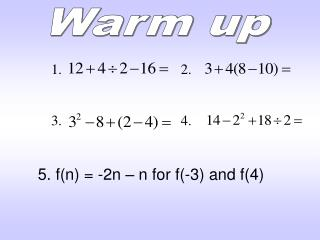DownloadDownload Presentation1. 2. 3. 4.

# 1. 2. 3. 4.

Download Presentation## 1. 2. 3. 4.

- - - - - - - - - - - - - - - - - - - - - - - - - - - E N D - - - - - - - - - - - - - - - - - - - - - - - - - - -
##### Presentation Transcript

1. Warm up • 1. 2. • 3. 4. 5. f(n) = -2n – n for f(-3) and f(4)

2. Math IDay 3 (8-17-11) UNIT QUESTION: How do we graph functions, and what can be done to change the way they look? Standard: MM1A1 Today’s Question: How do we find the domain and range of a function? Standard: MM1A1d

3. Domain and Range: Points Find the domain and range of the following: {(2, –3), (4, 6), (3, –1), (6, 6), (2, 3)} domain: {2, 3, 4, 6} range: {–3, –1, 3, 6}

4. Domain and Range: Notation • With continuous functions, we will use a notation called Set Notation using inequalities. • Examples: • x < 5 • 3 < x ≤ 9 Represented on the graph with a closed dot. Represented on the graph with an open dot.

5. Domain and Range: Graph Look horizontally: What x-values are contained in the graph? That’s your domain! Look vertically: What y-values are contained in the graph? That’s your range!

6. Domain and Range: Graph

7. Domain and Range: Graph

8. Domain and Range: Graph

9. Homework: Domain and Range Practice